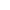# C/C++常见的输入函数总结

C++很多输入输出函数也可以引用C语言的，所以这边我们做一个总结：

## 输入函数

1、cin

• 最基本，也是最常用的方法，接收一个字符，整形或字符串
• 遇到回车，TAB或空格结束
``````#include <iostream>
using namespace std;
int main()
{
char a;
cin >> a;
cout << a << endl;
return 0;
}
``````

2、cin.get()

• 用法1：cin.get(字符变量名)，可以用来接收单个字符
``````#include <iostream>
using namespace std;
int main()
{
char ch;
ch = cin.get();
//也可以这样
//cin.get(ch); 效果一样
cout << ch;
return 0;
}
``````

• 用法2：cin.get(字符数组名，接收字符的数目)，可以用来接收一行字符串，可以包含空格
``````#include <iostream>
using namespace std;
int main()
{
char ch;
cin.get(ch,20);
cout << ch;
cout << endl;
return 0;
}
``````

3、cin.getline()

cin.getline(字符指针(char*),字符个数N(int)）

• 接收一行字符串，可以包含空格，与cin.get()用法2效果一样
• 主要适用于数组字符串，没有设置结束符默认以回车结束
• cin.getline()属于istream流
``````#include <iostream>
using namespace std;
int main()
{
char ch;
cin.getline(ch, 5);
cout << ch;
cout << endl;
return 0;
}
``````

4、getline()

istream& getline ( istream &is , string &str );

• 头文件：#include
• 用法：可以接收空格并输出，和cin.getline()类似，但cin.getline()属于istream流，getline()属于string流
• getline(cin,s)获取一行字符串。
``````// getline with strings
#include <iostream>
#include <string>
using namespace std;
int main () {
string str;
cout << "Please enter full name: ";
getline (cin,str);  //以回车为结束符
cout << "Thank you, " << str << ".\n";
}
``````

``````// getline with strings
#include <iostream>
#include <string>
using namespace std;
int main()
{
string str;
int a;
cin >> a;
cin.ignore();
getline(cin, str);
cout << str << endl;
return 0;
}
``````

geline（）这个函数坑比较多，多补充几个知识点，如若我们要多次输入多行的字符串，并打印到窗口，怎么做呢？

``````int main()
{
string str;
while (getline(cin, str))
{
cout << str << endl;
}
return 0;
}
``````

4、gets()

• 接受一个字符串，可以接收空格并输出，需包含“#include”
``````#include<iostream>
#include<string>
using namespace std;
int main()
{
char m;
gets(m);		//不能写成m=gets();
cout << m << endl;
}
``````

5、getchar()

• 接受一个字符,这个函数在标准C里面就有，在C++里也有，是getc()的宏定义
``````#include<iostream>
#include<string>
using namespace std;
int main ()
{
char ch;
ch = getchar(); //不能写成getchar(ch);
cout<<ch<<endl;
}
``````

C/C++毕竟是比较古老的东西，单单输入函数就有这么多种汕头电信 AI与大数据 税前12w 研究生